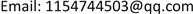1. 引言

2. 模型说明

3. 完全信息下的供应链博弈

1) 博弈初期，供应商和零售商观察市场需求，在完全信息供应商和零售商都能确切的了解到真实的市场需求 a i (在不完全信息下只有零售商可以观察到市场需求 a i ，供应商只能了解到市场需求均值 μ )；2) 完全信息下供应商基于真实的市场需求 a i 制定批发价格(不完全信息下供应商基于市场需求均值 μ 制定批发价格w)；3) 零售商R选择生产还是采购产品；4) 如果零售商选择自己生产，那么供应商和零售商基于自己对市场需求的了解同时决策产品数量，两者之间进行古诺竞争。若是零售商选择采购博弈进入下一阶段；5) 零售商R根据供应商提供的 { w } 和市场需求类型 a i ，决策其订货量q；6) 供应商根据零售商订货量q决策其销售数量Q，两者之间进行序贯博弈；7) 消费者购买，需求实现，双方完成销售和获得收益。

3.1. 生产和采购策略下的供应链博弈

Q M F = a i + c 3 , q M F = a i − 2 c 3

Π M F = ( a i + c ) 2 9 , π M F = ( a i − 2 c ) 2 9

Π = max [ a i − q − Q ] Q + w q ，由一阶条件可得供应商的销售量为 Q B F ( q ; a i ) = ( a i − q ) / 2 。零售商的利润表达式为 π = max [ a i − q − Q ( q ; a i ) − w ] q ，由一阶条件可得零售商的销售量为： q B F = ( a i − 2 w ) / 2 。将 q B F 代入 Q B F ( q ; a i ) 中得

Π B F = a i 2 + 12 a i w − 12 w 2 16 , π B F = ( a i − 2 w ) 2 8

Q M F = a i + c 3 , Π M F = ( a i + c ) 2 9 ; Q B F = a i + 2 w 4 , Π B F = a i 2 + 12 a i w − 12 w 2 16

q M F = a i − 2 c 3 , π M F = ( a i − 2 c ) 2 9 ; q B F = a i − 2 w 2 , π B F = ( a i − 2 w ) 2 8

3.2. 零售商的生产或采购决策

1) 当 c < a i / 14 时，供应商不愿意将产品出售给零售商，零售商只能自己生产；

2) 当 a i / 14 ≤ c < a i / 2 时，零售商以批发价格 w ¯ F = ( 3 a i − 2 2 ( a i − 2 c ) ) / 6 从供应商处采购产品；

3) 当 c ≥ a i / 2 时，零售商以批发价格 w F * = a i / 2 从供应商处采购产品。

4. 不完全信息下供应链博弈

4.1. 生产和采购策略下的供应链博弈

Q M A = μ + c 3 , q M A = 2 a i − μ − 2 c 3

Π M A = ( μ + c ) 2 9 , π M A = ( a i − 2 c ) ( 2 a i − μ − 2 c ) 9

Π = max [ μ − q − Q ] Q + w q ，由一阶条件可得供应商的销售量为 Q B A ( q ; μ ) = ( μ − q ) / 2 。零售商的利润表达式为 π = max [ a i − q − Q ( q ; μ ) − w ] q ，由一阶条件可得零售商的销售量为： q B A = ( 2 a i − μ − 2 w ) / 2 。将 q B A ( μ ) 代入 Q B A ( q ; μ ) 中得 Q B A = ( μ + 2 w ) / 4 。

Π B A = μ 2 + 12 μ w − 12 w 2 16 , π B A = ( 2 a i − μ − 2 w ) 2 8

Q M A = μ + c 3 ,; , Π B A = μ 2 + 12 μ w − 12 w 2 16

q M A = 2 a i − μ − 2 c 3 ,; q B A = 2 a i − μ − 2 w 2 , π B A = ( 2 a i − μ − 2 w ) 2 8

4.2. 零售商的生产或采购决策

π ¯ M A = ( μ − 2 c ) 2 9 , π ¯ B A = ( μ − 2 w ) 2 8

w ¯ A = 3 μ − 2 2 ( μ − 2 c ) 6

1) 当 c < μ / 14 时，供应商不愿意将产品出售给零售商；

2) 当 μ / 14 ≤ c < μ / 2 时，供应商制定批发价格 w ¯ A ；

3) 当 c ≥ μ / 2 时，供应商制定批发价格 w A * 。

π M A = ( a i − 2 c ) ( 2 a i − μ − 2 c ) 9 , π B A = ( 2 a i − μ − 2 w ) 2 8

w ¯ R A = 3 ( 2 a i − μ ) − 2 2 ( a i − 2 c ) ( 2 a i − μ − 2 c ) 6

1) 市场需求为高类型，

w ¯ H R A = 3 ( 2 a H − μ ) − 2 2 ( a H − 2 c ) ( 2 a H − μ − 2 c ) 6

2) 市场需求为低类型

w ¯ L R A = 3 ( 2 a L − μ ) − 2 2 ( a L − 2 c ) ( 2 a L − μ − 2 c ) 6

1) 当 0 < c < μ / 14 时，零售商自己生产产品(供应商不愿意将产品出售给零售商)；

2) 当 时，零售商采购产品，批发价格为 w ¯ A ；

3) 当 c ≥ μ / 2 时，零售商采购产品，批发价格为 w A * 。

5. 结论